Courses

# Pushdown Automata (PDA) Computer Science Engineering (CSE) Notes | EduRev

## Computer Science Engineering (CSE) : Pushdown Automata (PDA) Computer Science Engineering (CSE) Notes | EduRev

The document Pushdown Automata (PDA) Computer Science Engineering (CSE) Notes | EduRev is a part of the Computer Science Engineering (CSE) Course Theory of Computation.
All you need of Computer Science Engineering (CSE) at this link: Computer Science Engineering (CSE)

Part IV. Pushdown Automata (PDA)
As we learned, context free languages are generated using context free grammars. Context free languages are recognised using pushdown automata.
Following diagram shows a pushdown automation.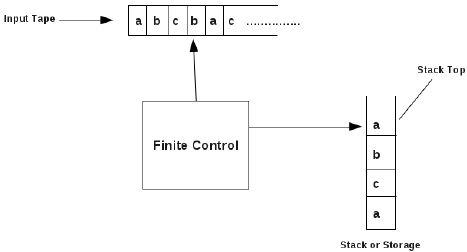The PDA has three components:
An input tape,
A control mechanism, and
A stack.
From the input tape, the finite control reads the input, and also
the finite control reads a symbol from the stack top.

6 Definition of PDA
A pushdown automation, P is a system,
P = (Q,∑, Γ, δ, q0, F)
where
Q is a set of states,
∑ is a set of input symbols,
Γ is a set of stack symbols (pushdown symbols),
q0 is the start state,
F is a set of final states,
δ is a transition function which maps,
(Q ×∑* ×Γ*) → (Q × Γ*)
The meaning of δ is explained below:
Consider a transition function, δ in a PDA defined by,
δ = (p, a, β) → (q, γ)
This means that
read a from the input tape,
pop the string β from stack top,
move from state p to state q,
push string γ on to the stack top.

Pushing the string abc on to the stack means,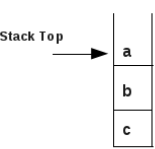Example:
Consider the following PDA,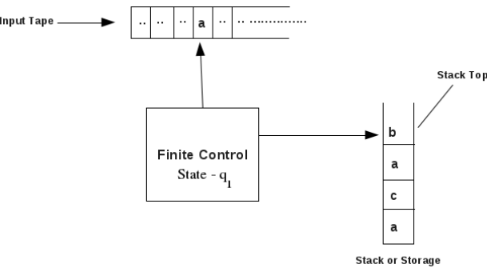Suppose PDA is currently in state qand the finite control points to state a and the stack contents are as shown above.
Let a transition is given as,
δ = (q1, a, b) → (q2, cd)
This means PDA currently in state q1, on reading the input symbol, a and popping b from the stack top changes to state q2 and pushes cd on to the stack top.
The new PDA is shown below: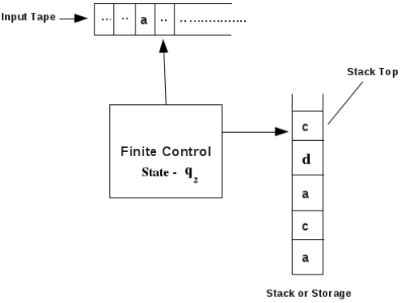7 Language Acceptability by PDA
Example 1:
Consider a PDA,
P = (Q,∑, Γ, δ, q0, F)
where
Q = {s, q, f}
∑= {a, b}
Γ = {a, b, c}
q0 = {s}
F = {f}
δ is given as follows:
1. (s, a, ε) → (q, a)
2. (s, b, ε) → (q, b)
3. (q, a, a) → (q, aa)
4. (q, b, b) → (q, bb)
5. (q, a, b) → (q, ε)
6. (q, b, a) → (q, ε)
7. (q, b, ε) → (q, b)
8. (q, ε, ε) → (f, ε)

Check whether the string abbbaaba is accepted by the above pushdown automation.
From the above, stack is initially empty, the start state of the PDA is s and PDA points to symbol, a as shown below: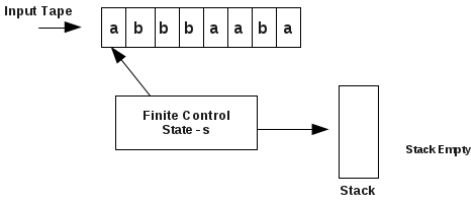1.
The PDA is in state s, stack top contains symbol ε. Consider the transition,
1. (s, a, ε) → (q, a)

This means PDA in state s, reads a from the tape, pops nothing from stack top, moves to state q and pushes a onto the stack.
PDA now is,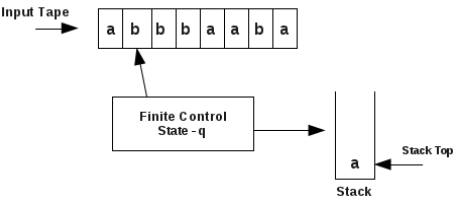2.
The PDA is in state q, finite control points to symbol b in the input tape, stack top contains symbol a. Consider the transition,
6. (q, b, a) → (q, ε)

This means PDA is in state q, reads b from the input tape, pops a from stack top, moves to state q and pushes nothing onto the stack.
PDA now is,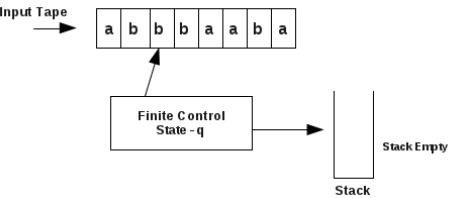3.
The PDA is in state q, finite control points to symbol b in the input tape, stack top contains symbol ε. Consider the transition,
7. (q, b, ε) → (q, b)

This means PDA is in state q, reads b from the input tape, pops ε from stack top, moves to state q and pushes b on to the stack.
PDA now is,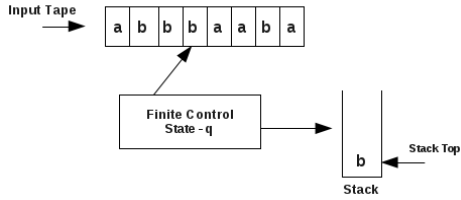4.
The PDA is in state q, finite control points to symbol b in the input tape, stack top contains symbol b. Consider the transition,
4. (q, b, b) → (q, bb)

This means PDA is in state q, reads b from the input tape, pops b from stack top, moves to state q and pushes bb onto the stack.
PDA now is,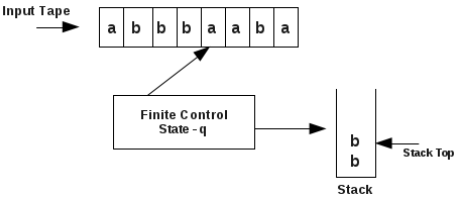5.
The PDA is in state q, finite control points to symbol a in the input tape, stack top contains symbol b. Consider the transition,
5. (q, a, b) → (q, ε)

This means PDA is in state q, reads a from the input tape, pops b from stack top, moves to state q and pushes ε on to the stack.
PDA now is,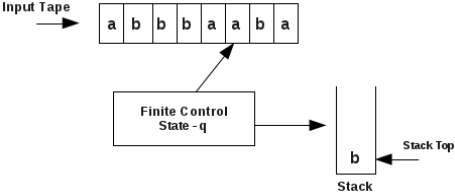6.
The PDA is in state q, finite control points to symbol a in the input tape, stack top contains symbol b. Consider the transition,
5. (q, a, b) → (q, ε)

This means PDA is in state q, reads a from the input tape, pops b from stack top, moves to state q and pushes nothing on to the stack.
PDA now is,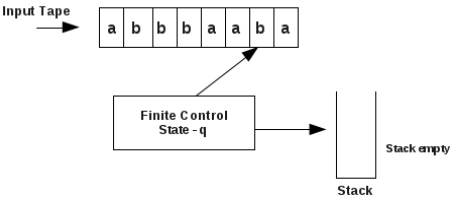7.
The PDA is in state q, finite control points to symbol b in the input tape, stack top contains symbol ε. Consider the transition,
7. (q, b, ε) → (q, b)

This means PDA is in state q, reads b from the input tape, pops ε from stack top, moves to state q and pushes b onto the stack.
PDA now is,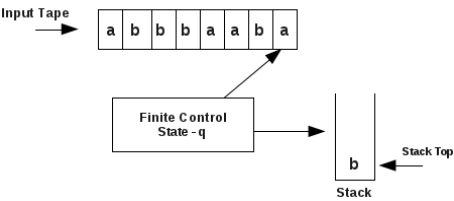8.
The PDA is in state q, finite control points to symbol a in the input tape, stack top contains symbol b. Consider the transition,
5. (q, a, b) → (q, ε)

This means PDA is in state q, reads a from the input tape, pops b from stack top, moves to state q and pushes ε on to the stack.
PDA now is,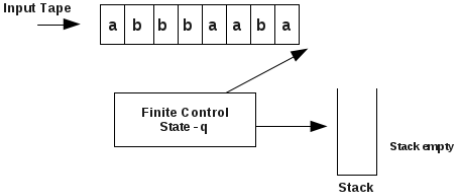9.
The PDA is in state q, finite control points to symbol ε in the input tape, stack top contains symbol ε. Consider the transition,
8. (q, ε, ε) → (f, ε)

This means PDA is in state q, reads ε from the input tape, pops ε from stack top, moves to state f and pushes nothing on to the stack.
PDA now is,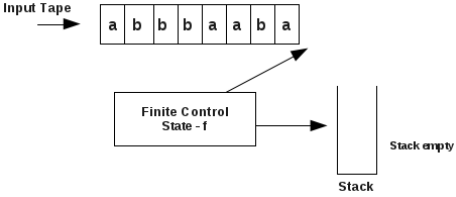Now PDA is in final state, f, and stack is empty. There are no more symbols in the input tape.
So the string abbbaaba is accepted by the above pushdown automation.

Example 2:
1.
Consider a PDA,
P = (Q,∑, Γ, δ, q0, F)
where
Q = {s, f}
∑= {a, b, c}
Γ = {a, b}
q0 = {s}
F = {f}
δ is given as follpws:
1. (s, a, ε) → (s, a)
2. (s, b, ε) → (s, b)
3. (s, c, ε) → (f, ε)
4. (f, a, a) → (f, ε)
5. (f, b, b) → (f, ε)

Check whether the string abacaba is accpeted by the above pushdown automation.

From the above, stack initially is empty, the start state of the PDA is s as shown below: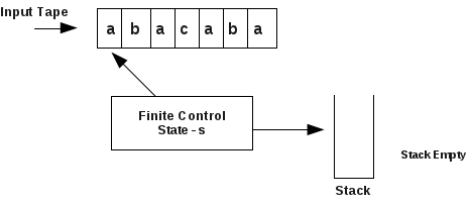1.
The PDA is in state s, finite control points to symbol a in the input tape, stack top contains symbol ε. Consider the transition,
1. (s, a, ε) → (s, a)

This means PDA is in state s, reads a from the input tape, pops nothing from stack top, moves to state s and pushes a on to the stack.
PDA now is,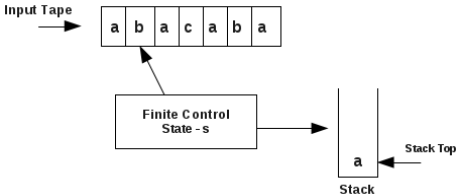2.
The PDA is in state s, finite control points to symbol b in the input tape, stack top contains symbol a. Consider the transition,
2. (s, b, ε) → (s, b)

This means PDA is in state s, reads b from the input tape, pops nothing from stack top, moves to state s and pushes b on to the stack.
PDA now is,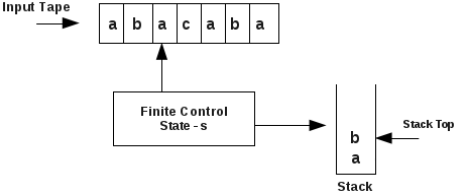3.
The PDA is in state s, finite control points to symbol a in the input tape, stack top contains symbol b. Consider the transition,
1. (s, a, ε) → (s, a)
This means PDA is in state s, reads a from the input tape, pops nothing from stack top, moves to state s and pushes a on to the stack.
PDA now is,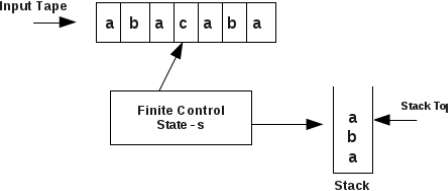4.
The PDA is in state s, finite control points to symbol c in the input tape, stack top contains symbol a. Consider the transition,
3. (s, c, ε) → (f, ε)

This means PDA is in state s, reads c from the input tape, pops nothing from stack top, moves to state f and pushes nothing onto the stack.
PDA now is,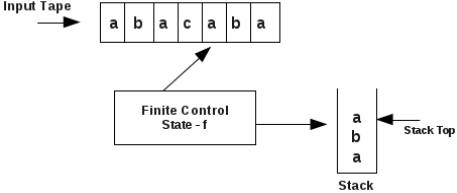5.
The PDA is in state f, finite control points to symbol a in the input tape, stack top contains symbol a. Consider the transition,
4. (f, a, a) → (f, ε)

This means PDA is in state f, reads a from the input tape, pops a from stack top, moves to state f and pushes nothing on to the stack.
PDA now is,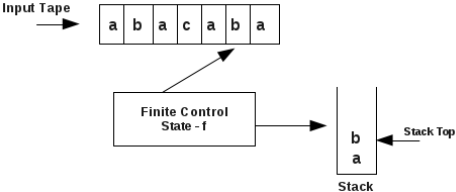6.
The PDA is in state f, finite control points to symbol b in the input tape, stack top contains symbol b. Consider the transition,
5. (f, b, b) → (f, ε)

This means PDA is in state f, reads b from the input tape, pops b from stack top, moves to state f and pushes nothing on to the stack.
PDA now is,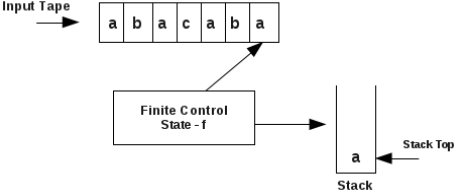7.
The PDA is in state f, finite control points to symbol a in the input tape, stack top contains symbol a. Consider the transition,
4. (f, a, a) → (f, ε)

This means PDA is in state f, reads a from the input tape, pops a from stack top, moves to state f and pushes nothing on to the stack.
PDA now is,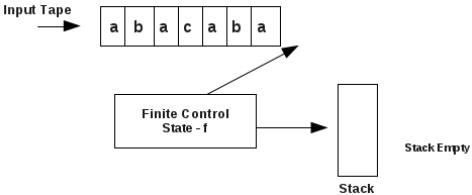Now there are no more symbols in the input string, stack is empty. PDA is in final state, f. So the string abacaba is accepted by the above pushdown automation.

Exercises:
1.
Consider a PDA,
P = (Q,∑, Γ, δ, q0, F)
where
Q = {q0, q1, q2, q3}
∑ = {0, 1}
Γ = {0, 1}
q0 = {q0}
F = {q3}
δ is given as follpws:
1. (q0, 0, ε) → (q1, 0)
2. (q1, 0, 0) → (q1, 00)
3. (q1, 1, 0) → (q2, ε)
4. (q2, 1, 0) → (q2, ε)
5. (q2, ε, ε) → (q3, ε)

Check whether the string 000111 is accpeted by the above pushdown automation.

2.
Consider a PDA,
P = (Q,∑, Γ, δ, q0, F)
where
Q = {q0, q1, q2, q3}
∑ = {a, b}
Γ = {a, b}
q0 = {q0}
F = {q3}
δ is given as follpws:
1. (q0, a, ε) → (q1, a)
2. (q1, a, a) → (q1, aa)
3. (q1, b, a) → (q2, a)
4. (q2, a, a) → (q2, ε)
5. (q2, ε, ε) → (q3, ε)

Check whether the string aabaa is accpeted by the above pushdown automation.

3.
Consider a PDA,
P = (Q,∑, Γ, δ, q0, F)
where
Q = {q0, q1, q2, q3}
∑ = {a, b}
Γ = {a, b}
q0 = {q0}
F = {q3}
δ is given as follpws:
1. (q0, a, ε) → (q1, a)
2. (q1, a, a) → (q1, aa)
3. (q1, b, a) → (q2, a)
4. (q2, b, a) → (q3, ε)
5. (q3, b, a) → (q2, a)
5. (q3, ε, ε) → (q4, ε)

Check whether the string aabbbb is accpeted by the above pushdown automation.

2.
Consider a PDA,
P = (Q,∑, Γ, δ, q0, F)
where
Q = {s, q, f}
∑ = {a, b}
Γ = {a, b}
q0 = {s}
F = {f}
δ is given as follpws:
1. (s, a, ε) → (s, a)
2. (s, b, ε) → (s, b)
3. (s, ε, ε) → (q, ε)
4. (q, a, a) → (q, ε)
4. (q, b, b) → (q, ε)
5. (q, ε, ε) → (f, ε)

Check whether the string aabbaa is accepted by the above pushdown automation.

8 Deterministic Pushdown Automata (DPDA)
A PDA is said to be deterministic, if
1. δ(q, a, b) contains at most one element, and
2. if δ(q, ε, b) is not empty, then
δ(q, c, b) must be empty for every input symbol, c.

For example, consider the following PDA,

Example 1:
Consider the PDA,
P = (Q,∑, Γ, δ, q0, F)
where
Q = {s, f}
∑ = {a, b, c}
Γ = {a, b}
q0 = {s}
F = {f}
δ is given as follpws:
1. (s, a, ε) → (s, a)
2. (s, b, ε) → (s, b)
3. (s, c, ε) → (f, ε)
4. (f, a, a) → (f, ε)
5. (f, b, b) → (f, ε)

Above is a deterministic pushdown automata (DPDA) because it satisfies both conditions of DPDA.

Example 2:
Consider the PDA,
P = (Q,∑, Γ, δ, q0, F)
where
Q = {s, f}
∑ = {a, b, c}
Γ = {a, b}
q0 = {s}
F = {f}
δ is given as follpws:
1. (s, a, ε) → (s, a)
2. (s, b, ε) → (s, b)
3. (s, c, ε) → (f, ε)
4. (f, a, a) → (f, ε)
5. (f, b, b) → (f, ε)

Above is a deterministic pushdown automata (DPDA) because it satisfies both conditions of DPDA.

9 Non-Deterministic Pushdown Automata (NPDA)
A PDA is said to be non- deterministic, if
1. δ(q, a, b) may contain multiple elements, or
2. if δ(q, ε, b) is not empty, then
δ(q, c, b) is not empty for some input symbol, c.

Example 1:
Consider the following PDA,
P = (Q,∑, Γ, δ, q0, F)
where
Q = {q0, q1, q2, q3}
∑ = {a, b}
Γ = {0, 1}
q0 = {q0}
F = {q3}
δ is given as follpws:
1. (q0, a, 0) → (q1, 10), (q3, ε)
2. (q0, ε, 0) → (q3, ε)
3. (q1, a, 1) → (q1, 11)
4. (q1, b, 1) → (q2, ε)
5. (q2, b, 1) → (q2, ε)
5. (q2, ε, 0) → (q3, ε)

Above is a non deterministic pushdown automata (NPDA).
Consider the transition 1, ie.

1. (q0, a, 0) → (q1, 10), (q3, ε)

Here (q0, a, 0) can go to q1 or q3. That this transition is not deterministic.

Example 2:
Consider the following PDA,
P = (Q,∑, Γ, δ, q0, F)
where
Q = {q0, qf }
∑= {a, b}
Γ = {0, 1}
q0 = {q0}
F = {qf }
δ is given as follpws:
1. (q0, ε, ε) → (qf , ε)
2. (q0, a, ε) → (q0, 0)
3. (q0, b, ε) → (q0, 1)
4. (q0, a, 0) → (q0, 00)
5. (q0, b, 0) → (q0, ε)
6. (q0, a, 1) → (q0, ε)
7. (q0, b, 1) → (q0, 11)

Above is a non deterministic pushdown automata (NPDA).
Consider the transitions, 1, 2 and 3.
1. (q0, ε, ε) → (qf , ε)
2. (q0, a, ε) → (q0, 0)
3. (q0, b, ε) → (q0, 1)
Here (q0, ε, ε) is not empty, also,
(q0, a, ε) and (q0, b, ε) are not empty.

Example 3:
Consider the following PDA,
P = (Q,∑, Γ, δ, q0, F)
where
Q = {s, p, q}
∑ = {a, b}
Γ = {a, b}
q0 = {s}
F = {q}
δ is given as follpws:
1. (s, a, ε) → (p, a)
2. (p, a, a) → (p, aa)
3. (p, a, ε) → (p, a)
4. (p, b, a) → (p, ε), (q, ε)

Above is a non deterministic pushdown automata (NPDA).
This is due to the transition,
4. (p, b, a) → (p, ε), (q, ε)

Example 4:
Consider the following PDA,
P = (Q,P, Γ, δ, q0, F)
where
Q = {q0, q1, q2, q3, f}
P = {a, b}
Γ = {a, b}
q0 = {q0}
F = {f}
δ is given as follpws:
1. (q0, a, ε) → (q1, a)
2. (q1, a, a) → (q1, a)
3. (q1, b, a) → (q2, ε)
4. (q2, b, a) → (q3, ε)
5. (q3, b, a) → (q2, ε)
6. (q2, ε, a) → (q2, ε)
7. (q2, ε, ε) → (f, ε)
8. (q1, ε, a) → (q1, ε)
9. (q1, ε, ε) → (f, ε)

Above is a non deterministic pushdown automata (NPDA).
This is due to the transitions, 6 and 4, ie,
6. (q2, ε, a) → (q2, ε)
4. (q2, b, a) → (q3, ε)
Also due to the transitions, 8 and 2 and 3, ie,
8. (q1, ε, a) → (q1, ε)
2. (q1, a, a) → (q1, a)
3. (q1, b, a) → (q2, ε)

10 Design of Pushdown Automata
For every CFG, there exists a pushdown automation that accepts it.
To design a pushdown automation corresponding to a CFG, following are the steps:
Step 1:
Let the start symbol of the CFG is S. Then a transition of PDA is,
δ(p, ε, ε) → (q, S)
Step 2:
For a production of the form, P → AaB, a transition of PDA is,
δ(q, ε, P) → (q, AaB)
For a production of the form, P → a, a transition of PDA is,
δ(q, ε, P) → (q, a)
For a production of the form, P → ε, a transition of PDA is,
δ(q, ε, P) → (q, ε)
Step 3:
For every terminal symbol, a in CFG, a transition of PDA is,
δ(q, a, a) → (q, ε)

Thus the PDA is,
P = (Q,∑, Γ, δ, q0, F)
where
Q = {p, q}
∑ = set of terminal symbols in the CFG
Γ =set of terminals and non-terminals in the CFG
q0 = p
F = q
δ is according to the above rules.

Example 1:
Consider the following CFG,
S → aA
A → aABC|bB|a
B → b
C → c
where S is the start symbol.
Design a pushdown automata corresponding to the above grammar.

Step 1:
Here start symbol of CFG is S. A transition is,
δ(p, ε, ε) → (q, S)

Step 3:
Consider the production, S → aA.
A transition is

δ(q, ε, S) → (q, aA)
Consider the production, A → aABC|bB|a.
Transitions are

δ(q, ε, A) → (q, aABC),
δ(q, ε, A) → (q, bB),
δ(q, ε, A) → (q, a).

Consider the production, B → b.
Corresponding transition is

δ(q, ε, B) → (q, b)

Consider the production, C → c
Corresponding transition is

δ(q, ε, C) → (q, c)

Step 3:
The terminal symbols in the CFG are, a, b, c.
Then the transitions are,

δ(q, a, a) → (q, ε)
δ(q, b, b) → (q, ε)
δ(q, c, c) → (q, ε)

Thus the PDA is,
P = (Q,∑, Γ, δ, q0, F)
where
Q = {p, q}
∑ = {a, b, c}
Γ = {S, A, B, C, a, b, c}
q0 = p
F = q
δ is given as follows:

δ(p, ε, ε) → (q, S)
δ(q, ε, S) → (q, aA)
δ(q, ε, A) → (q, aABC),
δ(q, ε, A) → (q, bB),
δ(q, ε, A) → (q, a).
δ(q, ε, B) → (q, b)
δ(q, ε, C) → (q, c)
δ(q, a, a) → (q, ε)
δ(q, b, b) → (q, ε)
δ(q, c, c) → (q, ε)

Example 2:
Consider the CFG,
S → AB|BC
A → aB|bA|a
B → bB|cC|b
C → c
where S is the start symbol.

Step 1:
Here start symbol of CFG is S. A transition is,
δ(p, ε, ε) → (q, S)

Step 3:
Consider the production, S → AB|BC
Transitions are

δ(q, ε, S) → (q, AB)
δ(q, ε, S) → (q, BC

Consider the production, A → aB|bA|a
Transitions are

δ(q, ε, A) → (q, aB),
δ(q, ε, A) → (q, bA),
δ(q, ε, A) → (q, a).
Consider the production, B → bB|cC|b
Transitions are

δ(q, ε, B) → (q, bB)
δ(q, ε, B) → (q, cC),
δ(q, ε, B) → (q, b)

Consider the production, C → c
Transitions are

δ(q, ε, C) → (q, c)

Step 3:
The terminal symbols in the CFG are, a, b, c.
Then the transitions are,

δ(q, a, a) → (q, ε)
δ(q, b, b) → (q, ε)
δ(q, c, c) → (q, ε)

Thus the PDA is,
P = (Q,∑, Γ, δ, q0, F)
where
Q = {p, q}
∑ = {a, b, c}
Γ = {S, A, B, C, a, b, c}
q0 = p
F = q
δ is given as follows:

δ(p, ε, ε) → (q, S)
δ(q, ε, S) → (q, AB)
δ(q, ε, S) → (q, BC)
δ(q, ε, A) → (q, aB)
δ(q, ε, A) → (q, bA)
δ(q, ε, A) → (q, a)
δ(q, ε, B) → (q, bB)
δ(q, ε, B) → (q, cC)
δ(q, ε, B) → (q, b)
δ(q, ε, C) → (q, c)
δ(q, a, a) → (q, ε)
δ(q, b, b) → (q, ε)
δ(q, c, c) → (q, ε)

Example 3:
Design a pushdown automata that accepts the language, L = {wcwR|w ∈ (a, b)*}
We learned earlier that the CFG corresponding to this language is,
S → aSa
S → bSb
S → c
where S is the start symbol.

Next we need to find the PDA corresponding to the above CFG.

Step 1:
Here start symbol of CFG is S. A transition is,
δ(p, ε, ε) → (q, S)

Step 3:
Consider the production, S → aSa
Transitions are

δ(q, ε, S) → (q, aSa)

Consider the production, S → bSb
Transitions are

δ(q, ε, S) → (q, bSb),

Consider the production, S → c
Transitions are

δ(q, ε, S) → (q, c)

Step 3:
The terminal symbols in the CFG are, a, b, c.
Then the transitions are,

δ(q, a, a) → (q, ε)
δ(q, b, b) → (q, ε)
δ(q, c, c) → (q, ε)

Thus the PDA is,
P = (Q,∑, Γ, δ, q0, F)
where
Q = {p, q}
∑ = {a, b, c}
Γ = {S, a, b, c}
q= p
F = q
δ is given as follows:

δ(p, ε, ε) → (q, S)
δ(q, ε, S) → (q, aSa)
δ(q, ε, S) → (q, bSb)
δ(q, ε, S) → (q, c)
δ(q, a, a) → (q, ε)
δ(q, b, b) → (q, ε)
δ(q, c, c) → (q, ε)

Example 4:
Design a pushdown automata that accepts the language, L = {w|w contains equal number of a’s and b’s } from the input alphabet {a,b}.

First, we need to find the CFG corresponding to this language. We learned in a previous section, the CFG corresponding to this is,
S → aSbS|bSaS|ε
where S is the start symbol.

Next we need to find the PDA corresponding to the above CFG.
Step 1:
Here start symbol of CFG is S. A transition is,
δ(p, ε, ε) → (q, S)

Step 3:
Consider the production, S → aSbS|bSaS|ε
Transitions are

δ(q, ε, S) → (q, aSbS)
δ(q, ε, S) → (q, bSaS)
δ(q, ε, S) → (q, ε)

Step 3:
The terminal symbols in the CFG are, a, b.
Then the transitions are,

δ(q, a, a) → (q, ε)
δ(q, b, b) → (q, ε)

Thus the PDA is,
P = (Q,∑, Γ, δ, q0, F)
where
Q = {p, q}
∑= {a, b}
Γ = {S, a, b}
q0 = p
F = q
δ is given as follows:

δ(p, ε, ε) → (q, S)
δ(q, ε, S) → (q, aSbS)
δ(q, ε, S) → (q, bSaS)
δ(q, ε, S) → (q, ε)
δ(q, a, a) → (q, ε)
δ(q, b, b) → (q, ε)

Example 5:
Design a pushdown automata that accepts the language corresponding to the regular expression, (a|b)*.

First, we need to find the CFG corresponding to this language. We learned in a previous section, the CFG corresponding
to this is,
S → aS|bS|a|b|ε
where S is the start symbol.

Next we need to find the PDA corresponding to the above CFG.
Step 1:
Here start symbol of CFG is S. A transition is,
δ(p, ε, ε) → (q, S)

Step 3:
Consider the production, S → aS|bS|a|b|ε
Transitions are

δ(q, ε, S) → (q, aS)
δ(q, ε, S) → (q, bS)
δ(q, ε, S) → (q, a)
δ(q, ε, S) → (q, b)
δ(q, ε, S) → (q, ε)

Step 3:
The terminal symbols in the CFG are, a, b.
Then the transitions are,

δ(q, a, a) → (q, ε)
δ(q, b, b) → (q, ε)

Thus the PDA is,
P = (Q,∑, Γ, δ, q0, F)
where
Q = {p, q}
∑ = {a, b}
Γ = {S, a, b}
q0 = p
F = q
δ is given as follows:

δ(p, ε, ε) → (q, S)
δ(q, ε, S) → (q, aS)
δ(q, ε, S) → (q, bS)
δ(q, ε, S) → (q, a)
δ(q, ε, S) → (q, b)
δ(q, ε, S) → (q, ε)
δ(q, a, a) → (q, ε)
δ(q, b, b) → (q, ε)

Exercises:
1. Design a pushdown automation to accept the language, L = {wwR|w ∈ {a, b}*}.
2. Design a pushdown automation to accept the language, L = {anbn|n > 0}.
3. Design a pushdown automation to accept the language, L = {anb2n|n > 0}.
4. Design a pushdown automation for the regular expression, r = 0*1*.
5. Design a pushdown automation to accept the language, L = {anbn+1|n = 1, 2, 3, ....}.
6. Design a pushdown automation to accept the language, L = {anbm|n > m ≥ 0}.
7. Construct PDA equivalent to the grammar S → aAA, A → aS/bS/a.
8. Construct PDA for the language L = {x ∈ (a, b)*/na(x) > nb(n)}.
9. Construct a PDA that accepts the language L = {anbam/n, m ≥ 1} by empty stack.

11 Applications of Pushdown Automata
An application of PDA is in parsing.
Parsing is the process of checking whether the syntax of a program is correct or not. For example, a PDA can be
constructed to check the syntax of all C programs.
Also, parsing is the process of checking whether a sentence written in a natural language is correct or not.
Parsing is of two types,
Top down parsing, and
Bottom up parsing.

Top Down Parsing
An example for top down parsing is given below:

Example 1:
Consider the following CFG,
S → aSb | ab
where S is the start symbol.
Check whether the syntax of string aabb is according to the above CFG.
Top down parsing is shown below: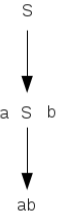Thus top down parsing is done beginning from the start symbol, by following a set of productions, reach the given string.
If the string cannot be reached, its syntax is not correct.
Above diagram is called a derivation tree (parse tree).
Here, by performing top down parsing , we got a2b2. So a2b2 belongs to the above CFL.

PDA for Top down Parsing
A top down parser can be implemented by using a Pushdown automation. Using the pushdown automation, we can parse
a given string.

Example 1:
For example, the PDA corresponding to the CFG, S → aSb | ab
is,
P = (Q,∑, Γ, δ, q0, F)
where
Q = {p, q}
∑= {a, b}
Γ = {S, a, b}
q0 = p
F = q
δ is given as follows:

δ(p, ε, ε) → (q, S)
δ(q, ε, S) → (q, aSb)
δ(q, ε, S) → (q, ab)
δ(q, a, a) → (q, ε)
δ(q, b, b) → (q, ε)
This PDA acts as a top down parser for the CFL corresponding to above CFG.

Bottom Up Parsing
This approach can also be used to check whether a string belongs to a context free language. Here, the string w is taken
and an inverted tree is made. This is done by performing a number of reductions starting from the given string using the
productions of the grammar.
Example 1:
Consider the grammar,
S → aAS|a|SS
A → SbA|ba
where S is the start symbol.
Check whether the string aabaa belongs to the CFL associated with the above grammar.

Bottom up parsing is shown below: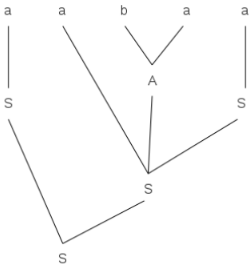Above is a bottom up parse tree.
Thus by performing a number of reductions, we finally reached the start symbol. So the string aabaa belongs to the
above CFL.
This parsing process can be done using a PDA.
PDA for Bottom down Parsing
A top down parser can be implemented by using a Pushdown automation. Using this pushdown automation, we can parse
a given string.

Example 1:
Consider the grammar,
S → aAS|a|SS
A → SbA|ba
where S is the start symbol.

A pushdown automation for performing bottom up parsing is shown below:
P = (Q,∑, Γ, δ, q0, F)
where
Q = {p, q}
∑ = {a, b}
Γ = {S, A, a, b}
q0 = p
F = q
δ is given as follows:

δ(q, ε, S) → (p, ε)
δ(q, ε, SAa) → (q, S)
δ(q, ε, a) → (q, S)
δ(q, ε, SS) → (q, S)
δ(q, ε, AbS) → (q, A)
δ(q, ε, ab) → (q, A)
δ(q, a, ε) → (q, a)
δ(q, b, ε) → (q, b)

This PDA acts as a bottom up parser for the CFL corresponding to above CFG.

Offer running on EduRev: Apply code STAYHOME200 to get INR 200 off on our premium plan EduRev Infinity!

## Theory of Computation

18 videos|44 docs|39 tests

,

,

,

,

,

,

,

,

,

,

,

,

,

,

,

,

,

,

,

,

,

;